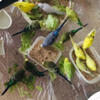## Welcome to the Treehouse Community

The Treehouse Community is a meeting place for developers, designers, and programmers of all backgrounds and skill levels to get support. Collaborate here on code errors or bugs that you need feedback on, or asking for an extra set of eyes on your latest project. Join thousands of Treehouse students and alumni in the community today. (Note: Only Treehouse students can comment or ask questions, but non-students are welcome to browse our conversations.)

### Looking to learn something new?

Treehouse offers a seven day free trial for new students. Get access to thousands of hours of content and a supportive community. Start your free trial today.# Python challenge: Create a function...

I tried my best for the ‘square’ part... but where does ‘number’ come in?

squaring.py
```def square(squared)
return (square * square)
print(square * 5)
```STAFF

The "number" is the parameter that goes into the parenthesis of the function. For example if you want to do a function that adds a number to itself you would write:

```def add(number):
return number + number
```

so then when you want to call the function, you have to pass in a number as an argument

```add(6)
```

Calling the function with the number argument is basically substituting the "number" parameter with 6 like so:

```def add(6)
return 6 + 6
```

Hopefully that helps!Your idea of the word square is totally off-track for this context. The word square, in this context, is referring to the function name. Which is `def square(number)`. Your idea of square (from what I observed) is that square will literally square the number, which is false. Look at this example;

```def NOT_SQUARE(parameter):
return parameter * parameter

>>> NOT_SQUARE(5)
25
```

The real magic is the Asterisk * symbol NOT the word square. The basic math rule is applied here where the Asterisk is multiplying the number (in this case parameter) by itself. Any number multiplied with itself gives you the square of that number.

If you're still confused, let me know.